Engineering Jobs   »   Mechincal Engineering quizs   »   Mechanical Engineering Quiz

# UPRVUNL’21 ME: Daily Practices Quiz. 8-Sep-2021

Quiz: Mechanical Engineering
Exam: UPRVUNL
Topic: Miscellaneous

Each question carries 1 mark
Negative marking: 1/4 mark
Time: 8 Minutes

Q1. If compression ratio is kept same then thermal efficiency of
(a) Otto cycle is less than Dual cycle
(b) Otto cycle is same as that for Diesel cycle
(c) Otto cycle is less than that of Diesel cycle
(d) Otto cycle is more than that of Diesel cycle

Q2. A 100 cc engine has the following parameter as 100 cc
(a) Cylinder volume
(b) Clearance volume
(c) Swept volume
(d) Full tank capacity

Q3. When heat is transferred from one particle of hot body to another by actual motion of the heated
particle, it is referred to as heat transfer by
(a) conduction
(b) convection
(d) conduction and convection

Q4. An ideal gas at 27°C is heated at constant pressure till its volume becomes two times. The temperature of gas then will be
(a) 81°C
(b) 900°C
(c) 600°C
(d) 327°C

Q5. The moment of inertia of a hollow circular section whose external diameter is 13 cm and internal diameter is 12 cm about centroidal axis is _______
(a) 437.5
(b) 137.5
(c) 284.5
(d) 384.1

Q6. Which cross-section of a cantilever beam which is loaded with UDL can give economical design–
(a) Square
(b) Circular
(c) I-Section
(d) Rectangular

Solutions
S1. Ans. (d)
Sol. For same compression ratio and heat addition, then thermal efficiency of otto cycle is more than that of Diesel cycle.

S2. Ans. (c)

S3. Ans. (a)

S4. Ans. (d)
Sol. At constant pressure. According to Charle’s law
V α T
V_1/V_2 =T_1/T_2
T_2=(V_2/V_1 ) T_1 [V_2=2V_1 ]
= 2T1 = 2(27 + 273)
T2 = 600 K = 327°C

S5. Ans. (d)
Sol. moment of inertia of follow circular section
I=π/64 ( d_O^4-di_O^4 )
=π/64 (13^4-12^4 )
=384.1 cm^4
S6. Ans. (c)
Sol. I-section of a cantilever beam which is loaded with UDL will give must economical design as for strongest cross section, moment of resistance (M_R) should be maximum, which depend upon section modules (2). For same cross-sectional area, section modules of I-section is maximum, hence it will be most economical design.

Sharing is caring!

•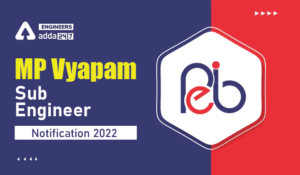MP Vyapam Sub Engineer Notification 2022...
•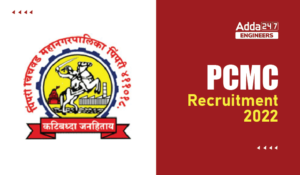PCMC Recruitment 2022, Check here the de...
•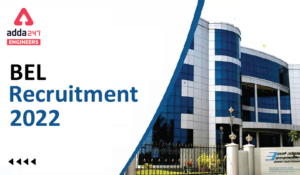BEL Recruitment 2022, Check here For 13 ...
•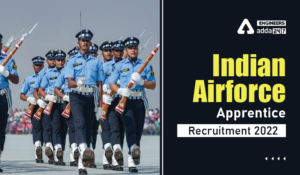Indian Air Force Apprentice Recruitment ...
•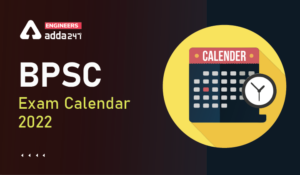BPSC Exam Calendar 2022, Check BPSC Assi...
•SSC Scientific Assistant IMD Recruitment...